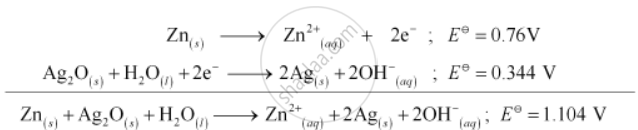# In the Button Cells Widely Used in Watches and Other Devices the Following Reaction Takes Place - Chemistry

In the button cells widely used in watches and other devices the following reaction takes place:

Zn(s) + Ag2O(s) + H2O(l) → Zn2+(aq) + 2Ag(s) + 2OH(aq)

Determine triangle_rG^Theta and E^Theta for the reaction.

#### Solution:. E^Theta = 1.104 V

We know that,

triangle_rG^Theta = -nFE^Theta

= −2 × 96487 × 1.04

= −213043.296 J

= −213.04 kJ

Concept: Batteries - Primary Batteries
Is there an error in this question or solution?
Chapter 3: Electrochemistry - Exercises [Page 91]

#### APPEARS IN

NCERT Class 12 Chemistry
Chapter 3 Electrochemistry
Exercises | Q 6 | Page 91

Share